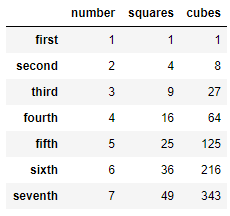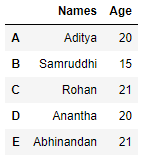# Create a DataFrame from a Numpy array and specify the index column and column headers

Let us see how to create a DataFrame from a Numpy array. We will also learn how to specify the index and the column headers of the DataFrame.

Approach :

1. Import the `Pandas `and `Numpy `modules.
2. Create a `Numpy `array.
3. Create list of index values and column values for the DataFrame.
4. Create the DataFrame.
5. Display the DataFrame.

Example 1 :

 `# importiong the modules ` `import` `pandas as pd ` `import` `numpy as np ` ` `  `# creating the Numpy array ` `array ``=` `np.array([[``1``, ``1``, ``1``], [``2``, ``4``, ``8``], [``3``, ``9``, ``27``],  ` `                  ``[``4``, ``16``, ``64``], [``5``, ``25``, ``125``], [``6``, ``36``, ``216``],  ` `                  ``[``7``, ``49``, ``343``]]) ` ` `  `# creating a list of index names ` `index_values ``=` `[``'first'``, ``'second'``, ``'third'``, ` `                ``'fourth'``, ``'fifth'``, ``'sixth'``, ``'seventh'``] ` `  `  `# creating a list of column names ` `column_values ``=` `[``'number'``, ``'squares'``, ``'cubes'``] ` ` `  `# creating the dataframe ` `df ``=` `pd.DataFrame(data ``=` `array,  ` `                  ``index ``=` `index_values,  ` `                  ``columns ``=` `column_values) ` ` `  `# displaying the dataframe ` `print``(df) `

Output :Example 2 :

 `# importiong the modules ` `import` `pandas as pd ` `import` `numpy as np ` ` `  `# creating the Numpy array ` `array ``=` `np.array([[``'Aditya'``, ``20``], [``'Samruddhi'``, ``15``], ` `                  ``[``'Rohan'``, ``21``], [``'Anantha'``, ``20``],  ` `                  ``[``'Abhinandan'``, ``21``]]) ` ` `  `# creating a list of index names ` `index_values ``=` `[``'A'``, ``'B'``, ``'C'``, ``'D'``, ``'E'``] ` `  `  `# creating a list of column names ` `column_values ``=` `[``'Names'``, ``'Age'``] ` ` `  `# creating the dataframe ` `df ``=` `pd.DataFrame(data ``=` `array,  ` `                  ``index ``=` `index_values,  ` `                  ``columns ``=` `column_values) ` ` `  `# displaying the dataframe ` `print``(df) `

Output :Example 3 :

 `# importiong the modules ` `import` `pandas as pd ` `import` `numpy as np ` ` `  `# creating the Numpy array ` `array ``=` `np.array([[``'CEO'``, ``20``, ``5``], [``'CTO'``, ``22``, ``4.5``],  ` `                  ``[``'CFO'``, ``21``, ``3``], [``'CMO'``, ``24``, ``2``]]) ` ` `  `# creating a list of index names ` `index_values ``=` `[``1``, ``2``, ``3``, ``4``] ` `  `  `# creating a list of column names ` `column_values ``=` `[``'Names'``, ``'Age'``,  ` `                 ``'Net worth in Millions'``] ` ` `  `# creating the dataframe ` `df ``=` `pd.DataFrame(data ``=` `array,  ` `                  ``index ``=` `index_values,  ` `                  ``columns ``=` `column_values) ` ` `  `# displaying the dataframe ` `print``(df) `

Output :My Personal Notes arrow_drop_upCheck out this Author's contributed articles.

If you like GeeksforGeeks and would like to contribute, you can also write an article using contribute.geeksforgeeks.org or mail your article to contribute@geeksforgeeks.org. See your article appearing on the GeeksforGeeks main page and help other Geeks.

Please Improve this article if you find anything incorrect by clicking on the "Improve Article" button below.

Article Tags :

Be the First to upvote.

Please write to us at contribute@geeksforgeeks.org to report any issue with the above content.